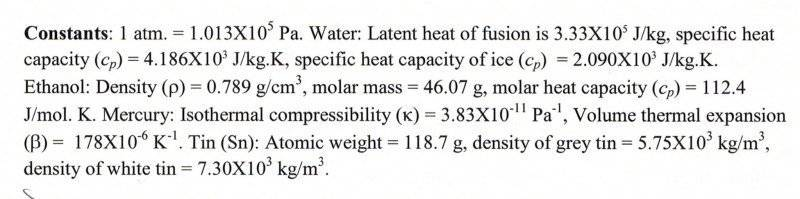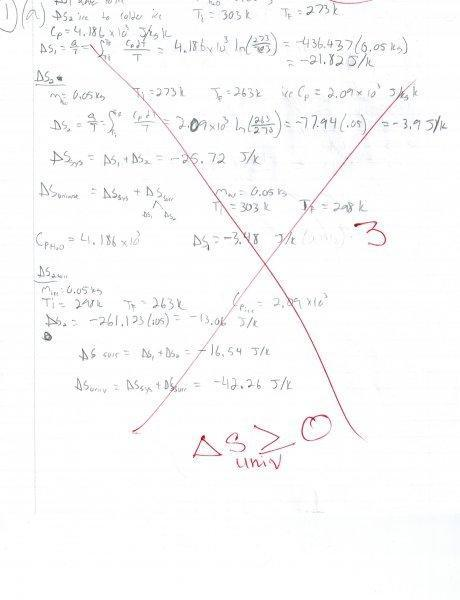# Change in entropy of system and universe

## Homework Statement

50.0g of water (the system) at 30C is frozen to ice at a final temp of -10C in a freezer. Assuming that the volume of water remains the same during the process, calculate the change in entropy of the system and the change of entropy of the thermal universe when the system reaches thermal equilibrium.## Homework Equations

ΔS=Q/T=∫Ti..Tf(CpdT)/T

## The Attempt at a Solution

See attached image for attempt at solution. I already missed the question in class and just want to know how to properly do it at this point. Thanks.rude man
Homework Helper
Gold Member

## Homework Statement

50.0g of water (the system) at 30C is frozen to ice at a final temp of -10C in a freezer. Assuming that the volume of water remains the same during the process, calculate the change in entropy of the system and the change of entropy of the thermal universe when the system reaches thermal equilibrium.

## Homework Equations

ΔS=Q/T=∫Ti..Tf(CpdT)/T
This is correct for the entropy change for the water. What about the ice part?

Andrew Mason
Homework Helper

## Homework Statement

50.0g of water (the system) at 30C is frozen to ice at a final temp of -10C in a freezer. Assuming that the volume of water remains the same during the process, calculate the change in entropy of the system and the change of entropy of the thermal universe when the system reaches thermal equilibrium.
View attachment 75716

## Homework Equations

ΔS=Q/T=∫Ti..Tf(CpdT)

In order to calculate the change in entropy of the freezer (i.e the surroundings) assume that the freezer is so large that its temperature does not change during the process. In that case, the change in entropy is -Q/Tfreezer where Q is the heat flow out of the water. What is the heat flow out of the water?

AM